# RD Sharma Class 9 and Chapter Algebraic Identities Maths Solutions

## Maths Class 9 and Chapter Algebraic Identities RD Sharma SolutionsFilter
Filters :
CLASSES
•
•
•
•
•
•
•
CHAPTERS
•
• 15 More

# RD Sharma Class 9 Maths Chapter 4 Algebraic Identities

The word Algebra itself indicates the usage of alphabets among the numbers and Algebra forms an interesting division of Mathematics. Algebra finds its place in solving equations that are used in maths and also in other subjects like physics and chemistry. Hence forming an important part of the subject Maths. The students, by now, must be aware of the basics of Algebra like the algebraic expressions. Algebraic Identities takes the learners into the next step of learning Algebra. Now through this unit, let us look into the next chapter of Algebra called ‘Algebraic Identities.’  Learning and understanding algebra is very easy unless the students have the right study materials and guidance.

Talking of the right study materials, it is important that the reference maths textbook used by the students must cover all the important topics that come under a particular chapter. Coming under the category of most widely referred maths textbooks in India is RD Sharma’s owing to the simple approaches used to solve the problems. RD Sharma’s books have been a preferred textbook for many years now by the students studying the CBSE syllabus. These books come on par with the latest CBSE prescribes syllabus and cover all the details required by the students to face the examination. RD Sharma’s books have also become an optimum choice for the students aiming to crack the competitive entrance examinations such as IIT JEE, VITEEE, etc. This is because apart from covering all the relevant and necessary concepts, RD Sharma’s books also methodically break even the complex concepts into an easily understandable format.

And now talking of the right guide, it is essential to take help from a trusted solution guide for the reference textbook used as this tends to save a lot of learning time of the students. Oftentimes the students in the process of solving the exercise problems by themselves may land up in confusion. They might not be aware of the right formula or simple methods to approach the problems and hence often land up wasting a lot of time. The online solution guide like the Doubtnut eliminates such uncertainties and time-waste among the learners. Any doubts that occur during the process of solving textbook problems can easily be erased with the help of doubtnut.com. All the solutions are worked out in an exercise-wise format and the students can easily track the solutions. RD Sharma Class 9 Solutions Chapter 4 Algebraic Identities, found on Doubtnut is going to provide all that is necessary for the students to excel in learning the unit.

Algebraic Identities Class 9 Maths RD Sharma Solutions

In the previous classes, the students must have studied the chapter of Algebraic expressions and by now would have a fair idea about solving the algebraic equations. This unit, Algebraic Identities too, involves solving of various equations but using a certain algebraic formula called the Algebraic Identities. So far, the students must have encountered with the monomial and binomial equations that are easier to arrive at a solution. But when there are polynomial equations, solving them is not going to be an easy task and that is when these algebraic identities play a key role.

According to the NCERT textbook, the definition of Algebraic Identities goes,

“Algebraic identity is an algebraic equation that is true for all values of the variables occurring in it”.

Basically, an identity is nothing, but an expression that comes in the form of LHS and RHS. An algebraic equation, where there would be equality between the LHS and RHS and that holds true for all the values of variables.

For example, let us consider one of the standard identities, a+b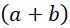2 = a2 + 2ab + b2. Here ‘a’ and ‘b’ are the variables. Let us substitute the value of ‘a’ as 2 and the value of ‘b’ as 3. Applying it to the identity,

(2+3)2 = 22 + (2*2*3) + 32

52 = 4 + 12 + 9

25 = 25

LHS = RHS

Here we can see that the LHS is equalised to RHS by substituting the values for the variables. Hence, this equation, a+b2 = a2 + 2ab + b2, can be called an identity. Through this current chapter, the students are going to witness more such Algebraic Identities. There are going to be problems based on these algebraic identities. And hence it is going to be a necessity for the students to remember a lot of such standard algebraic identities which can be applied to the problems in order to solve.

## What is the purpose of learning algebraic identities?

Algebraic Identities form one of the core units of the Algebra. These identities find their place not just in the subject of mathematics, but also in other subjects like physics. The basics formulae learnt right now in the form of identities is going to be carried along throughout a student’s educational journey.

The algebraic identities are generally used when the factorisation of polynomial equations is done. A lot of polynomial expressions becomes really difficult to solve and this is where factorisation is done. For example, the expression x2+x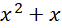can be factorised into x(x+1). And this expression is of a binomial. But when it comes to polynomial, the factorisation gets more difficult and is done with the help of the algebraic identities. These algebraic identities are helpful when simplifying the equations by reverse-factorisation techniques as well. For example, 4x2+6xy+9y2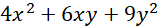takes the form of a2+2ab+b2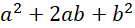, where a = 2x and b = 3y. Now, we can say that the equation can hence be written in the form, (4x + 3y)2. This will be very useful in further classes when solving the quadratic equations and other related applications.

So, apply this concept to multiply huge numbers, too. Let us consider multiplying we need to multiply 910x1090. This is going to be a big task and time consuming as well. But then, we can just try to factorise these two multiples to make them simpler. We know that 910 can be written as (1000-90) and the number 1090 can be written as (1000+90). Applying the same to the question, it takes the form of (1000 – 90) x (1000 + 90). This can be further be simplified using the identity,a+b×a-b=a2- b2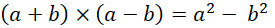. These are some of the examples that demonstrate the role these Algebraic Equations play.

Topics and Subtopics of RD Sharma Class 9 Maths Chapter 4 Algebraic Identities

RD Sharma Class 9 Maths Chapter 4 Algebraic Identities covers a lot of basic concepts that are carried over in the further higher classes, too.  Learning and remembering all these identities are crucial in order to dive further into Algebra. Before learning to solve the textbook problems, it is important that the learners are aware of the topics and subtopics of the chapter covered in the textbook. This helps the students be aware of the textbook content and would be very useful during the learning process. Knowing the topics and subtopics in a unit helps them to plan their study time-table in a more efficient way.

Let us know the topics and subtopics of RD Sharma Class 9 Maths Chapter 4 Algebraic Identities based on which the solutions are rolled out on doubtnut.com:

### Explaining Identities

Here the introduction to what are algebraic identities is given here along with suitable demonstration.

### Standard Identities

The derivation of the standard identities that are widely used when solving algebraic equations is demonstrated.

### Applying Identities

The application of the standard identities when solving the related problems are demonstrated with examples. The exercise problems are widely based on this subtopic.

Since it is important to be aware of the standard identities. Here is a list of the identities based on which the exercise problems are framed in RD Sharma textbook:

a+b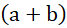2 = a2 + 2ab + b2

a+b2 = a2 + 2ab + b2

a2- b2=a+b ×(a-b)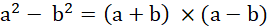(a + b)= a3 + b3 + 3ab (a + b)

(a - b)= a3 - b3 - 3ab (a - b)

a3 + b3 = (a + b) (a2 + b2 – ab)

a3 – b3 = (a – b) (a2 + b2 – ab)

(x + y +z)2=x2 +y2 + z2+2xy+2yz+2x

It can be said that there is a lot of things to remember in this unit and also learning the skill of applying the right identity at the right place. With the help of the systematically worked-out video solutions found on doubtnut.com, the whole process of learning this unit becomes easier. The students can experience a classroom kind of experience as an expert teacher takes them through each solution step-by-step. The doubts can be clarified from the comfort of the homes, at any time, by just posting the photo of the question. All exam-oriented tension can be avoided and the students turn more focussed through ‘studying through watching the lessons’ rather than the conventional method of’ studying through reading’.